Fractals etc.

The following fractal generators all use JavaScript and an html canvas element to create their images.

The multibrot generator: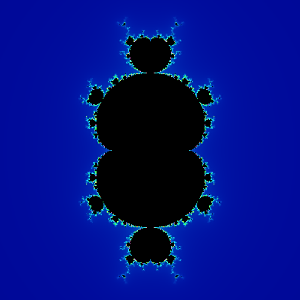The icon generator:The Latööcarfian dream generator: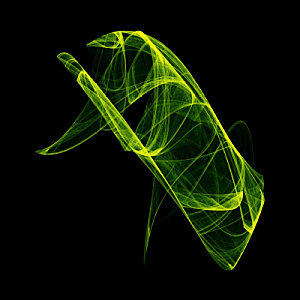The Zaslavsky web map generator: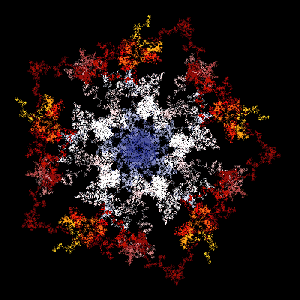Using a JavaScript complex number class to draw multibrot sets and icon attractors

Most online Mandelbrot generators use real-number equivalents of the complex number functions, partly because, for degree two, it is easier, and also because it is likely to be faster.  However, higher degrees require the use of complex polynomial equations.  The multibrot and icon generators above demonstrate the use of a JavaScript complex number class to perform the calculations.  The multibrot script iterates the following for the complex numbers c and z:

f(z) = zn + c

```c = new cx(x, y);
z = new cx(0, 0);

z = cx.add(cx.pow(z, n), c);
```

where the degree n is an integer greater than or equal to two.

The icon attractors are based on equations described in Symmetry in Chaos(for the FTC:  “As an Amazon Associate I earn from qualifying purchases.”) by Michael Field and Martin Golubitsky.  They were produced by iterating functions like the one below for the complex number z:

f(z) = [λ + αzz̄ + βRe(zn) + ωi]z + γn-1

```with (cx) {

z = new cx(x, y);

a = mult(mult(alpha, z), conj(z));
b = mult(beta, pow(z, n).re);
o = mult(omega, i);
g = mult(gamma, pow(conj(z), n-1));

}
```

JavaScript classes are part of the ECMAScript 2015 (ES6) standard.  You will need an up-to-date version of just about any browser except Internet Explorer, which is no longer supporte by its manufacturer.

Here is the complex number class definition and reference.  If you use it, a link to russellcottrell.com would be nice.

Gallery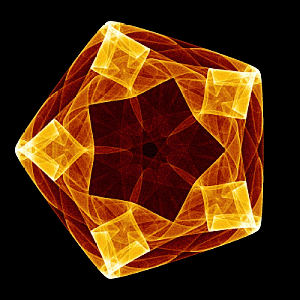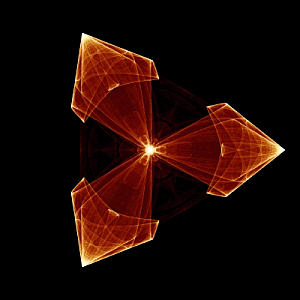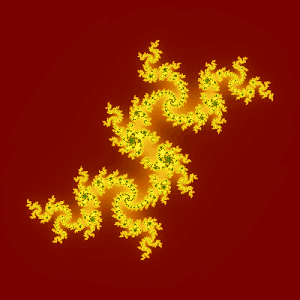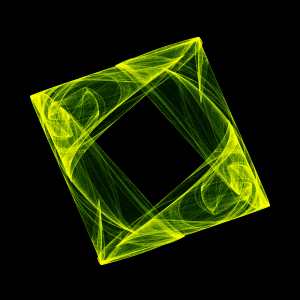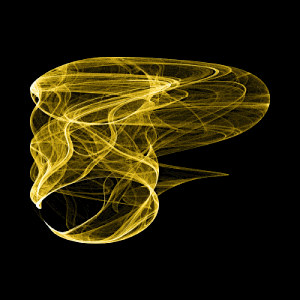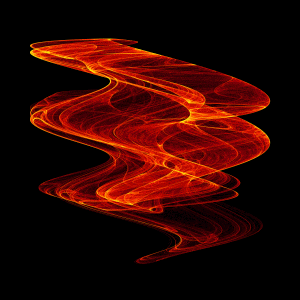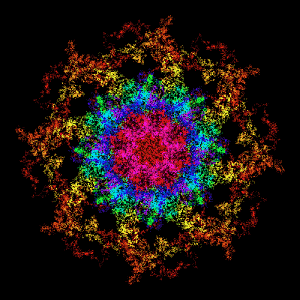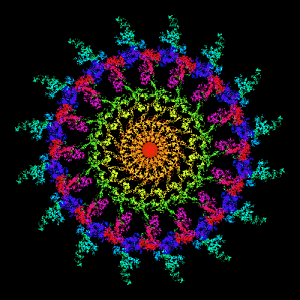Mandala Explorer

Back in 2007 I made the fractal program Mandala Explorer.  It is a little dated but it still works.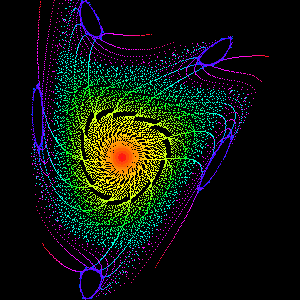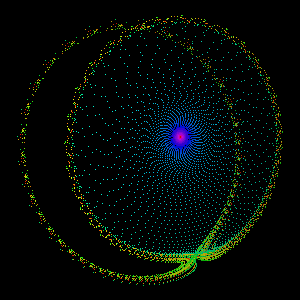Miscellany

Chaos theory is a branch of mathematics focusing on the study of states of dynamical systems whose apparently-random states of disorder and irregularities are often governed by deterministic laws that are highly sensitive to initial conditions.  Chaos theory is an interdisciplinary theory stating that, within the apparent randomness of chaotic complex systems, there are underlying patterns, constant feedback loops, repetition, self-similarity, fractals, and self-organization.

Chaotic behavior exists in many natural systems, including fluid flow, heartbeat irregularities, weather and climate.  It also occurs spontaneously in some systems with artificial components, such as stock market and road traffic.

Self-organization is a process where some form of overall order arises from local interactions between parts of an initially disordered system.  The process can be spontaneous when sufficient energy is available, not needing control by any external agent.  It is often triggered by seemingly random fluctuations, amplified by positive feedback.

Self-organization occurs in many physical, chemical, biological, robotic, and cognitive systems.  Examples of self-organization include crystallization, thermal convection of fluids, chemical oscillation, animal swarming, neural circuits, and artificial neural networks.

A fractal is a subset of a Euclidean space for which the fractal dimension strictly exceeds the topological dimension.

Fractals appear the same at different levels, as illustrated in successive magnifications of the Mandelbrot set

Fractals are of particular relevance in the field of chaos theory, since the graphs of most chaotic processes are fractals.

An attractor is a set of numerical values toward which a system tends to evolve, for a wide variety of starting conditions of the system.

An attractor is called strange if it has a fractal structure.

If a strange attractor is chaotic, exhibiting sensitive dependence on initial conditions, then any two arbitrarily close alternative initial points on the attractor, after any of various numbers of iterations, will lead to points that are arbitrarily far apart (subject to the confines of the attractor), and after any of various other numbers of iterations will lead to points that are arbitrarily close together.

A complex number is a number that can be expressed in the form a + bi, where a and b are real numbers, and i is a solution of the equation x2 = -1.  Because no real number satisfies this equation, i is called an imaginary number.  For the complex number a + bi, a is called the real part, and b is called the imaginary part.

Complex numbers extend the concept of the one-dimensional number line to the two-dimensional complex plane by using the horizontal axis for the real part and the vertical axis for the imaginary part.  The complex number a + bi can be identified with the point (a, b) in the complex plane.

In electrical engineering, the Fourier transform is used to analyze varying voltages and currents.  The treatment of resistors, capacitors, and inductors can then be unified by introducing imaginary, frequency-dependent resistances for the latter two and combining all three in a single complex number called the impedance.

In fluid dynamics, complex functions are used to describe potential flow in two dimensions.

The complex number field is intrinsic to the mathematical formulations of quantum mechanics, where complex Hilbert spaces provide the context for one such formulation that is convenient and perhaps most standard.  The original foundation formulas of quantum mechanics – the Schrödinger equation and Heisenberg's matrix mechanics – make use of complex numbers.

In special and general relativity, some formulas for the metric on spacetime become simpler if one takes the time component of the spacetime continuum to be imaginary.  (This approach is no longer standard in classical relativity, but is used in an essential way in quantum field theory.)

russellcottrell.com